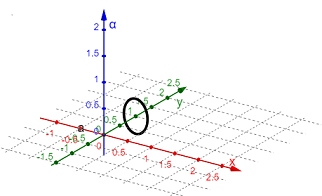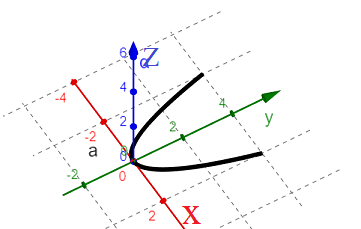# Sketch or describe the curves for the vector functions below. (a) r(t)=1/4 cos (t)i+j+1/3sin(t)k...

## Question:

Sketch or describe the curves for the vector functions below.

(a) {eq}\vec r(t)=\frac{1}{4} cos (t) \vec i+ \vec j+\frac{1}{3} sin(t) \vec k{/eq}

(b) {eq}\vec r(t)=t \vec i+t^2 \vec j+0 \vec k{/eq}

## Graph of Parametric Curve:

A parametric curve in space is described by the position vector

{eq}\vec r(t) = x(t) \vec i + y(t) \vec j + z(t) \vec k {/eq}

with t being the parameter.

The graph of the parametric curve is produced by plotting the sequence of points (x,y,z)

as the parameter t varies.

(a) A sketch of the parametric curve described by the vector function

{eq}\displaystyle \vec r(t)=\frac{1}{4} cos (t) \vec i+ \vec j+\frac{1}{3} sin(t) \vec k {/eq}

is produced plotting the points (x,y,z) as the parameter t varies and is shown in the figure below.

The curve is a circle in the plane y=1.(b) The parametric curve

{eq}\vec r(t)=t \vec i+t^2 \vec j+0 \vec k {/eq}

is a parabola in the plane z=0 and its sketch is illustrated in the figure below.